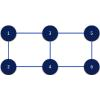Physical Review Letters ( IF 8.385 ) Pub Date : 2020-06-29 , DOI: 10.1103/physrevlett.125.010501
Suguru Endo; Jinzhao Sun; Ying Li; Simon C. Benjamin; Xiao YuanVariational quantum algorithms have been proposed to solve static and dynamic problems of closed many-body quantum systems. Here we investigate variational quantum simulation of three general types of tasks—generalized time evolution with a non-Hermitian Hamiltonian, linear algebra problems, and open quantum system dynamics. The algorithm for generalized time evolution provides a unified framework for variational quantum simulation. In particular, we show its application in solving linear systems of equations and matrix-vector multiplications by converting these algebraic problems into generalized time evolution. Meanwhile, assuming a tensor product structure of the matrices, we also propose another variational approach for these two tasks by combining variational real and imaginary time evolution. Finally, we introduce variational quantum simulation for open system dynamics. We variationally implement the stochastic Schrödinger equation, which consists of dissipative evolution and stochastic jump processes. We numerically test the algorithm with a 6-qubit 2D transverse field Ising model under dissipation.

down
wechat
bug